# Topology Optimization: Complance Minimization using SESO with Bilinear Square Element

London Journal of Engineering Research
Volume | Issue | Compilation
Authored by Hélio Luiz Simonetti , Valério Silva Almeida, Francisco de Assis das Neves
Classification: NA
Keywords: topology optimization, compliant mechanisms, SESO.
Language: English

This paper presents the Smoothing-ESO (SESO) technique for topology optimization of structures implemented in MATLAB using 4-node bilinear square elements. The lines comprising this code include definition of design domain, finite element analysis, sensitivity analysis, mesh-independency filter, optimization algorithm. Extensions and changes in the algorithm are also included in order to solve multiple load cases and compliant mechanisms design. In addition, a comparison is made with other optimization methods as Bi-directional Evolutionary Structural Optimization (BESO), Sequential Element Rejection and Admission (SERA) and Solid Isotropic Material with Penalization (SIMP). Thus, numerical examples are presented to demonstrate the ability of proposed methods to solve topology optimization problems.

# Hélio Luiz Simonettiα, Valério Silva Almeidaσ,& Francisco de Assis das Nevesρ

## __________________________________________

### ABSTRACT

This paper presents the Smoothing-ESO (SESO) technique for topology optimization of structures implemented in MATLAB using 4-node bilinear square elements. The lines comprising this code include definition of design domain, finite element analysis, sensitivity analysis, mesh-independency filter, optimization algorithm. Extensions and changes in the algorithm are also included in order to solve multiple load cases and compliant mechanisms design. In addition, a comparison is made with other optimization methods as Bi-directional Evolutionary Structural Optimization (BESO), Sequential Element Rejection and Admission (SERA) and Solid Isotropic Material with Penalization (SIMP). Thus, numerical examples are presented to demonstrate the ability of proposed methods to solve topology optimization problems.

### Keywords:topology optimization, compliant mechanisms, SESO.

Author α: Department of Mathematics, Federal Institute of Minas Gerais (IFMG).

σ: School of Engineering of the University of São Paulo (EPUSP).

ρ:  Department of Civil Engineering, Federal University of Ouro Preto (UFOP).

1. ### INTRODUCTION

Evolutionary structural optimization (ESO) method was firstly introduced by Xie and Steven (1993). The idea is based on a simple and empirical concept that a structure evolves towards an optimum by slowly removing elements with lowest stresses. Chu et al. (1996) to maximize the stiffness of the structure, stress criterion was replaced with elemental strain energy criterion. Bi-directional evolutionary structural optimization (BESO) by Querin et al. (1998, 2000) method is an extension of that idea which allows for new elements to be added in the locations next to those elements with highest stresses. Rozvany and Querin proposed some improvements of this method under the term SERA (Sequential Element Rejection and Admission) where a “virtual material” was introduced, without the use of any intermediate densities or power law interpolations Rozvany and Querin (2004). Recently Matlab codes with extensions to Pareto strategies by Suresh (2010) and  a methodology for ground structure based topology optimization in arbitrary 2D and 3D domains have been implemented using Matlab, Zegard and Paulino (2014, 2015). Three- dimensional topology optimization codes can be found in the work presented by Liu and Tovar (2014).

The present paper use of a Matlab program that incorporates the strategies for topology optimization based on the SESO technique. The proposed code is very similar to the 99-line by Sigmund (2001) except for the material update subroutine, where the optimality criterion has been replaced by the SESO algorithm. This program can be effectively used in personal computers for educational purposes of engineering students  interested in the field of topology optimization, as an educational tool in courses on topology optimization.

### 2.1  Finite Element Model

The works of Sigmund (2001) and Andreassen et al. (2011) use the SIMP model to perform topology optimization considering the isotropic material. The SESO technique was implemented in this article is based in the proposals by these authors. Thus, the calculations for isotropic material form are made in the finite element stiffness matrix formulation. The finite element used in this formulation is the 4-node bilinear square element. The stiffness matrix of this element results from the following double integration: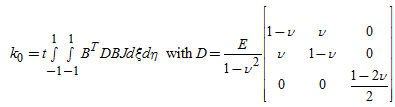(1)

In which: t is the thickness of the element. Since it is done in 2D, t = 1. D Is the constitutive relation.is the Young’s modulus of the isotropic material,is the Poisson’s ratio of the isotropic material. The [B] matrix is given as follows for this element: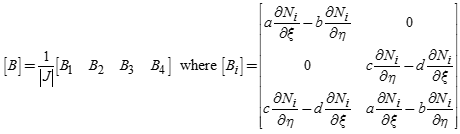(2)

The parameters a, b, c and d are given by: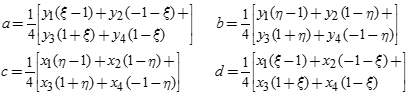(3)

And the shape derivatives ofare given by: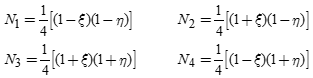(4)

J is the determinant given by: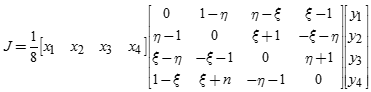(5)

### 2.2  Compliance Optimization Problem

The topology optimization problem for maximum stiffness structural design is defined as the minimization of the compliance, where the objective is to find the material distribution that minimizes the structure’s deformation under the prescribed support and loading conditions, subjected to a volume constraint.  In the SESO technique, a structure is optimized by removing p% and returning (1-p%) of the elements, that is, the element itself, instead of its associated physical or material parameters, is treated as the design variable. Therefore, to minimize compliance by removing elements, it is clear that the most effective way is to eliminate those elements having the lowest values so that the increase in compliance is minimal. Thus, taking into account the equilibrium, problem can be written as at equation 6. Whereis the global displacement,is the global stiffness matrix,andare the element displacement vector and stiffness matrix, respectively, NE is the number of elements used to discretize the design domain, KU = F is the equilibrium equation F is the vector of the applied loads in the structure, xi is the design variable of the i-th element and X is the vector of the design variables.

The global stiffness matrixis assembled from the element stiffness matrices, which are obtained multiplying the element isotropic stiffness matrixby xi of the i-th element, since Young’s moduli are assumed to depend linearly on the variable, i.e.,. These design variables are discrete in the SESO technique, so variable xi can only be zero or one. Nevertheless, in order to avoid obtaining a singular stiffness matrix, a non-zero lower bound is assigned toas shown in 6.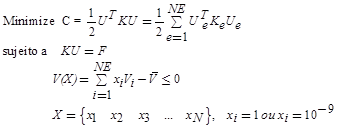(6)

1. ### COMPARING SESO WITH OTHER TOPOLOGY OPTIMIZATION METHODS WITH A MESH-INDEPENDENCY FILTER

The SESO algorithm will be compared to the ESO, SERA, Soft-Kill BESO and SIMP algorithms for this comparison it should be noted that the filter scheme is a heuristic technique for overcoming the checkerboard and mesh dependency problems in topology optimization. Therefore, it is better to compare the algorithms at two levels-one without the mesh-independency filter and the other with it. A long cantilever shown in figure 1 is selected as a test example because it involves a series of bars broken during the evolutionary procedure of the topology optimization. A concentrated load F = 1.0 N is applied downward in the middle of the free end. Young’s modulus E = 1.0 MPa and Poisson’s ratio v = 0.3 are assumed. The design domain is discretized with 160 × 40 four node plane stress elements.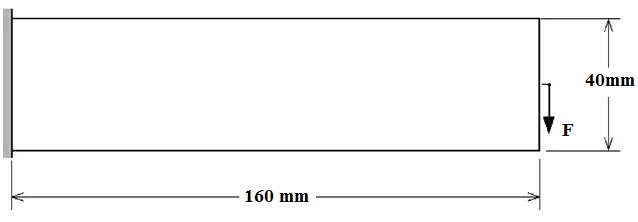Figure 1: Design domain

Table 1 lists the used parameters and solutions obtained of the various topology optimization algorithms.  It can be seen that the topology optimization algorithms produce very similar topologies except that the SIMP design has some grey areas of intermediate material densities. The topologies presented in Table 1 present cleaner definitions of the members and are more conducive to practical use.

Table 1: Comparison of topology optimization methods with a mesh-independency filter

 Optimizationparameters Totaliteration Solutions Compliance SESO ER=0.02 100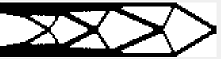418,5 SERA PR=0.03SR=1.15 97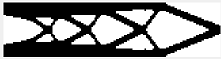365.46 Soft-Kill BESO ER=0.0125P=3.0 80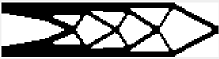196.41 SIMP move=0.2p=3 153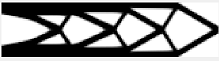376.30

All presented methods show similar topologies. However, the topologies of the SESO, SIMP and soft-kill BESO methods are very similar. Although the soft-kill SESO converges with fewer iterations than SESO and SIMP, the lowest computational cost is due to SESO, however, SESO methods is the one that has the highest value for mean compliance in the optimal location analyzed.

### 4.1  EXAMPLE 1 - Compliant Mechanisms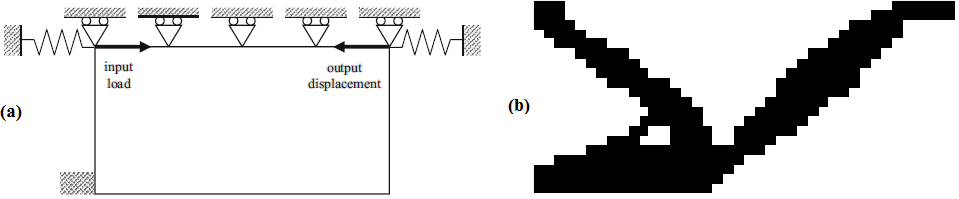Figure 2: (a) Design domain and (b) Optimal topology

### 4.2  EXAMPLE 2 – MBB BEAM

The SESO method, with quadrilateral elements, was used to optimize the so-called MBB beam, which has been extensively studied in topology optimization, see Figure 3. The load is applied vertically in the upper left corner and there is a symmetric boundary condition along the left edge and the structure is supported horizontally in the lower right corner. The beam’s dimensions are 60 × 20 elements and correspond to half of the structure. The volume fraction limit is 50% and two different filter radii were used: 1.5 and 3.5. Figure 4a and 4b show the optimum configurations obtained with the present formulation.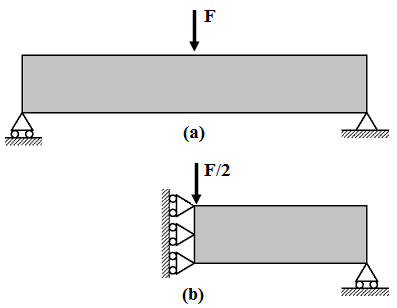Figure 3:  Design model for the topology optimization of the MBB-beam

###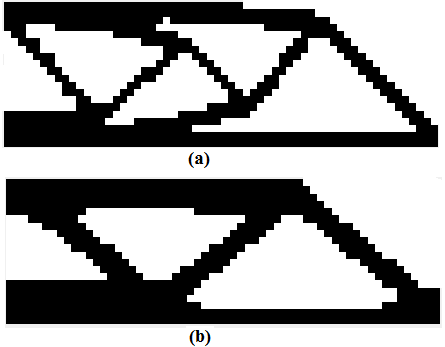Figure 4: Topologies for the MBB-beam with different filtering

(a) Filter 1.5 and (b) Filter 3.5

### 4.3  EXAMPLE 3 – MULTIPLE LOAD CASES

The SESO method was extended for compliance minimization in solve multiple load cases topology optimization problems. If two load cases are considered, force and displacement vectors must become two-column vectors. The objective function should be defined as the sum of compliances for each load case. Figure 5a shows the design domain and the boundary conditions of the proposed problem to cantilever beam. Figure 5b shows the optimum design for the cantilever when topology is optimized corresponds to the two-load case. It can be noticed that obtained topologies agree well with the solutions published in the paper by Sigmund (2001) using the SIMP method.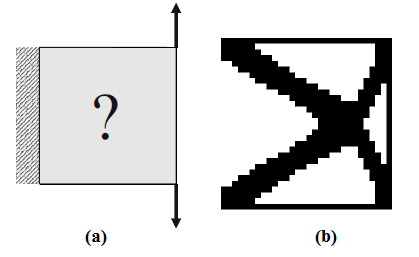Figure 5: (a) Design domain and (b) Optimal topology

### V.    CONCLUSÕES

This paper presents SESO technique in code Matlab for minimum compliance problems and its extension to multiple load cases and compliant mechanism design. The code uses four-node bilinear square elements and the results presented with the numerical examples analyzed have shown that the SESO technique is efficient and robust in problems of plane elasticity.

### ACKNOWLEDGEMENTS

The authors are grateful for the total support by grant #2016/02327-5, São Paulo Research Foundation (FAPESP) for the funding provided to the present research.

### REFERENCES

1. Chu DN, Xie YM, Hira A, Steven GP (1996) Evolutionary structural optimization for problems with stiffness constraints. Finite Elem Anal Des 21:239–251.
2. Liu K, Tovar A (2014) An efficient 3D topology optimization code written in Matlab. Struct Multidiscip Optim 50:1175–1196.
3. Querin OM, Steven GP, Xie YM (1998) Evolutionary structural optimization (ESO) using a bi-directional algorithm. Eng Comput 15:1031–1048.
4. Querin OM, Young V, Steven GP, Xie YM (2000) Computational efficiency and validation of bi-directional evolutionary structural optimization. Comput Methods Appl Mech Eng 189: 559–573.
5. Rozvany GIN, Querin O (2004) Sequential element rejections and admissions (SERA) method: applications to multi constraint problems. In: Proceedings of the 10th AIAA/ ISSMO Multidisc. Anal. Optim. Conference, Albany, New York.
6. Saxena A, Ananthasuresh G (2000) On an optimal property of compliant topologies. Struct Multidiscip Optim 19:36–49
7. Sigmund O (2001) A 99 line topology optimization code written in Matlab. Struct Multidiscip Optim 21:120–127.
8. Suresh K (2010) A 199-line matlab code for pareto-optimal tracing in topology optimization. Struct Multidiscip Optim 42: 665–679.
9. Xie YM, Steven GP (1993) A simple evolutionary procedure for structural optimization. Comput Struct 49:885–896.
10. Zegard T, Paulino GH (2014) GRAND - ground structure based topology optimization for arbitrary 2D domains using MATLAB. Struct Multidiscip Optim 50:861–882.
11. Zegard T, Paulino GH (2015) GRAND3 - ground structure based topology optimization for arbitrary 3D domains using MATLAB. Struct Multidiscip Optim 52:1161–1184.#### For Authors

/symposiums/webinars, networking opportunities, and privileged benefits.
Authors may submit research manuscript or paper without being an existing member of LJP. Once a non-member author submits a research paper he/she becomes a part of "Provisional Author Membership".

Know more#### For Institutions

Society flourish when two institutions come together." Organizations, research institutes, and universities can join LJP Subscription membership or privileged "Fellow Membership" membership facilitating researchers to publish their work with us, become peer reviewers and join us on Advisory Board.

Know more#### For Subscribers

Subscribe to distinguished STM (scientific, technical, and medical) publisher. Subscription membership is available for individuals universities and institutions (print & online). Subscribers can access journals from our libraries, published in different formats like Printed Hardcopy, Interactive PDFs, EPUBs, eBooks, indexable documents and the author managed dynamic live web page articles, LaTeX, PDFs etc.

Know more